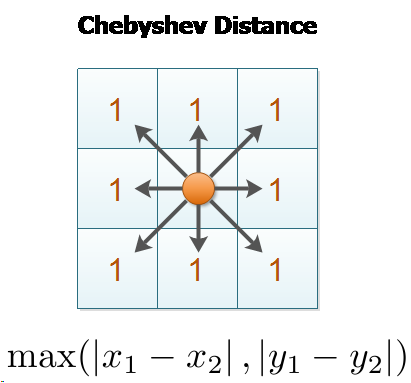×

Search anything:

# Chebyshev distance

#### similarity measurement chebyshev distance Algorithms Software EngineeringGet this book -> Problems on Array: For Interviews and Competitive Programming

Chebyshev distance is a distance metric which is the maximum absolute distance in one dimension of two N dimensional points. It has real world applications in Chess, Warehouse logistics and many other fields.

It is, also, known as Tchebychev distance, maximum metric, chessboard distance and L∞ metric.

It is named after Pafnuty Chebyshev, a Russian mathematician who is the recipient of the Demidov Prize and is known for his work on probability, statistics, mechanics, analytical geometry and number theory.

The concept of Chebyshev distance is captured by this image:### Properties

Properties of Chebyshev distance are:

• The two dimensional Manhattan distance also has circles in the form of squares, with sides of length √2r, oriented at an angle of π/4 (45°) to the coordinate axes, so the planar Chebyshev distance can be viewed as equivalent by rotation and scaling to the planar Manhattan distance

• A sphere formed using the Chebyshev distance as a metric is a cube with each face perpendicular to one of the coordinate axes, but a sphere formed using Manhattan distance is an octahedron: these are dual polyhedra, but among cubes, only the square (and 1-dimensional line segment) are self-dual polytopes

### Example

Consider two points P1 and P2 with co-ordinates as follows:

``````
P1 = (p1, p2, ..., pN)
P2 = (q1, q2, ..., qN)
``````

The chebyshev distance between the two points P1 and P2 is:

``````
Chebyshev distance = MAXIMUM (|pi - qi|) where i is 1 to N
``````

### Applications

Applications of Chebyshev distance are:

• Chess: The minimum number of moves needed by a king to go from one square on a chessboard to another equals the Chebyshev distance between the centers of the squares

• Warehouse logistics: The Chebyshev distance is sometimes used in warehouse logistics as it effectively measures the time an overhead crane takes to move an object#### OpenGenus Tech Review Team

The official account of OpenGenus's Technical Review Team. This team review all technical articles and incorporates peer feedback. The team consist of experts in the leading domains of Computing.